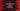# 3 different C programs to print a half diamond star pattern## C program to print a half diamond star pattern:

This post will show you how to print a half diamond pattern using star in C programming language. The program will take the number of rows of the pattern as an input from the user and it will print that pattern. We will learn how to use for loop and while loop to print the pattern.

### Algorithm to print a half diamond star pattern:

Let me show you how it looks like.

``````*
**
***
****
***
**
*

*
**
***
****
*****
****
***
**
*``````

The first one is a half diamond star pattern of height 4 and the second one is a half diamond star pattern of height 5.

We will use the below algorithm to print the half diamond pattern:

• Take the height of the pattern as an input from the user.
• Run one loop for height * 2 - 1 number of time.
• Inside this loop, run another loop to print each row of the pattern. It will print one star for the first row, two stars for the second row etc. i.e. the total count of stars will keep increasing. Once it reaches the height of the pattern as entered by the user, it will keep decreasing the total count of stars.
• At the end of each row, print a newline character to move to the next line.

### Example 1: C program to print a half diamond star pattern by using for loops:

The below C program uses two for loops to print a half diamond star pattern by taking the height of the pattern as an input from the user:

``````#include <stdio.h>

int main()
{
int height, totalStar = 1;

printf("Enter the height of the pattern: ");
scanf("%d", &height);

for (int i = 1; i < height * 2; i++)
{
for (int j = 1; j <= totalStar; j++)
{
printf("*");
}
printf("\n");

if (i < height)
{
totalStar++;
}
else
{
totalStar--;
}
}
}``````

Here,

• height is an integer variable to hold the height of the pattern and *totalStar is another integer variable to keep the value of total number of stars to print for a specific row.
• It asks the user to enter the height of the pattern. This value is assigned to the totalStar variable.
• The for loop runs from 1 to height * 2 - 1.
• On each iteration of the loop, it runs another loop to print the stars. This loops runs for totalStar number of times.
• At the end of each iteration, it prints a new line to move the control to the next line.
• If the value of i is smaller than height it increments the totalStar value by 1. Else, it decrements the totalStar value by 1.

If you run this program, it will print output as like below:

``````Enter the height of the pattern: 4
*
**
***
****
***
**
*

Enter the height of the pattern: 5
*
**
***
****
*****
****
***
**
*``````

As you can see here, it prints the pattern for any value of height.

### Example 2: C program to print a half diamond star pattern by using while loops:

Let’s use while loops to write this program. These loops will work in a similar way. The values of i and j will be initialized before the loops starts and the increment will be done at the end of each iteration of the loop. We will not change any other logical parts of the program.

``````#include <stdio.h>

int main()
{
int height, totalStar = 1, i = 1, j;

printf("Enter the height of the pattern: ");
scanf("%d", &height);

while (i < height * 2)
{
j = 1;
while (j <= totalStar)
{
printf("*");
j++;
}
printf("\n");

if (i < height)
{
totalStar++;
}
else
{
totalStar--;
}
i++;
}
}``````

As you can see here, the values of i and j are initialized before the loop starts. The star is printed in the loop and the values of i or j are incremented by 1.

It will print similar patterns.

### Example 3: C program to print a half diamond star pattern by using a separate function:

Let’s use a separate function to print the pattern. This function will take the height of the pattern as its parameter and it will print the pattern. We can use for loops or while loops to print it as shown in the previous examples. Let me show you with for loops and a separate function:

``````#include <stdio.h>

void printHalfDiamondStarPattern(int height)
{
int totalStar = 1;
for (int i = 1; i < height * 2; i++)
{
for (int j = 1; j <= totalStar; j++)
{
printf("*");
}
printf("\n");

if (i < height)
{
totalStar++;
}
else
{
totalStar--;
}
}
}

int main()
{
int height;

printf("Enter the height of the pattern: ");
scanf("%d", &height);

printHalfDiamondStarPattern(height);
}``````
• We created a new function printHalfDiamondStarPattern to print the pattern. It takes the height of the pattern as its parameter.
• It uses for loops to print the pattern. Since this function doesn’t return any value, the return type is void.

You will get similar output if you run it.